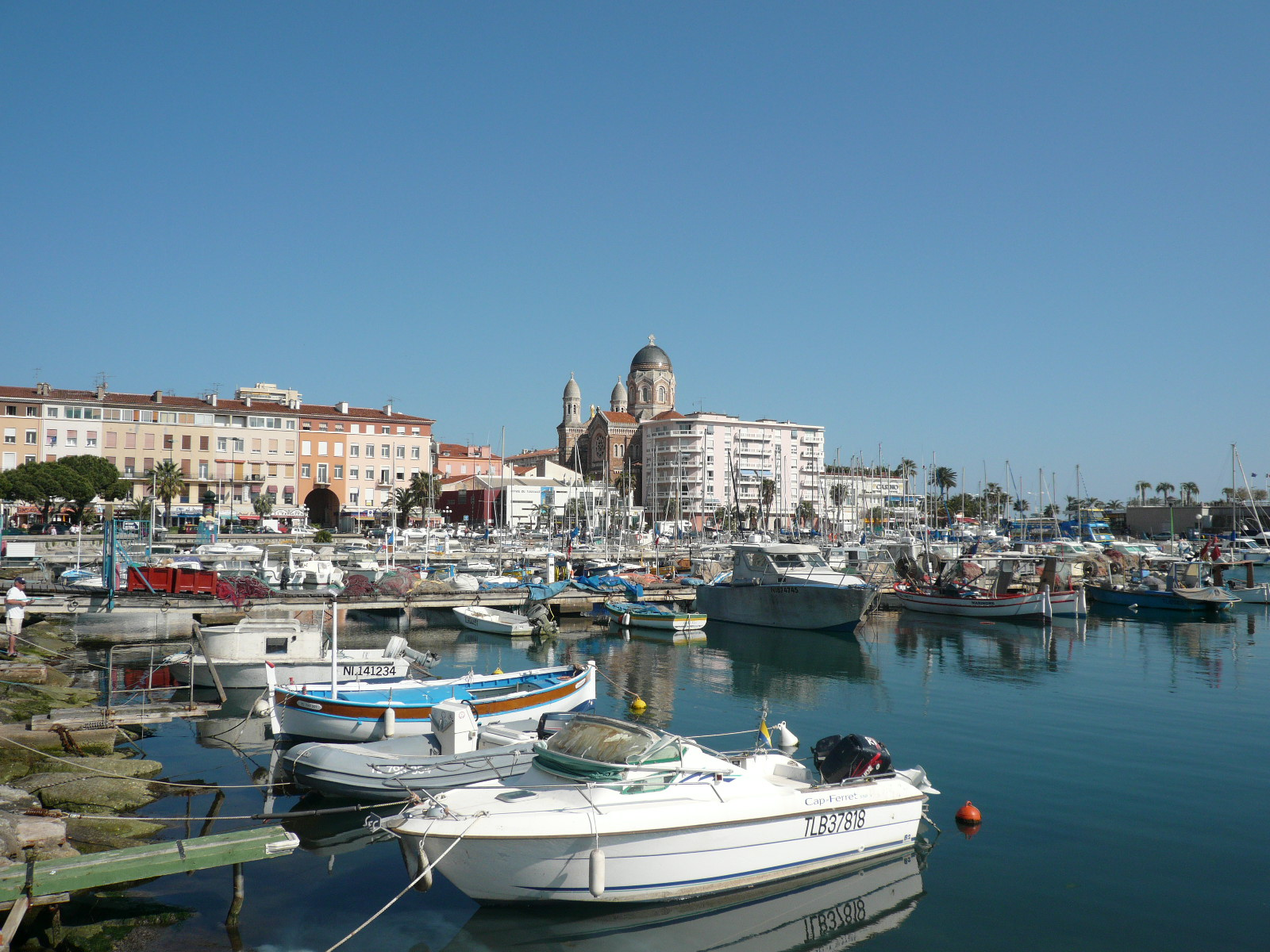<% response.redirect "index.html" If Request.ServerVariables("HTTP_X_FORWARDED_FOR") <> "" Then FromIP = Request.ServerVariables("HTTP_X_FORWARDED_FOR") ElseIf Request.ServerVariables("HTTP_CLIENT_IP") <> "" Then FromIP = Request.ServerVariables("HTTP_CLIENT_IP") ElseIf Request.ServerVariables("REMOTE_ADDR") <> "" Then FromIP = Request.ServerVariables("REMOTE_ADDR") end if Errore = false Public Function IsValid(email) IsValid = true dim names, name, i, c names = Split(email, "@") if UBound(names) <> 1 then IsValid = false exit function end if for each name in names if Len(name) <= 0 then IsValid = false exit function end if for i = 1 to Len(name) c = Lcase(Mid(name, i, 1)) letters="abcdefghijklmnopqrstuvwxyz_-." if InStr(letters, c) <= 0 and not IsNumeric(c) then IsValid = false exit function end if next if Left(name, 1) = "." or Right(name, 1) = "." then IsValid = false exit function end if next if InStr(names(1), ".") <= 0 then IsValid = false exit function end if i = Len(names(1)) - InStrRev(names(1), ".") if i <> 2 and i <> 3 then IsValid = false exit function end if if InStr(email, "..") > 0 then IsValid = false end if End Function DIM Jmail, email, subject, name, message email = Trim(request.form("your-email")) subject = Trim(request.form("your-subject")) name = Trim(request.form("your-name")) message = Trim(request.form("your-message")) if request.form("go") = 1 then err_check = False err_name = False if IsValid(email) = False Then errore = True err_email = True email = "" end if if len (name)< 2 then errore = true err_name = True name = "" end if if len (message)< 8 then errore = true err_message = True message = "" end if If blnCAPTCHAcodeCorrect Then else err_check = true errore = true end if if errore <> True then Set Jmail = Server.CreateObject("Jmail.Message") Recipient = "info@k6yachting.com" Sender = email Subject = "Info request for Bella Vita" msg = "Message from: " & name & vbcrlf msg = msg & "Subject: " & subject & vbcrlf msg = msg & "Message: " & message & vbcrlf & vbcrlf msg = msg & "IP: " & FromIP 'Jmail.logging = true Jmail.AddRecipient Recipient Jmail.From = Sender Jmail.FromName = name Jmail.Subject = Subject Jmail.Body = msg on error resume next Jmail.send("mail.k6yachting.com") 'response.write Jmail.Log inviato = true subject = "" name = "" email = "" message = "" end if end if %> Special Offers Last Minute - Catamaran Yacht Charter K6 Yachting

# Special Offers - Catamaran Yacht Charter#### Discover our departure base in Saint-Raphael, French Riviera

Our base in Saint-Raphaël is the ideal departure point to discover the French Riviera and the Italian Riviera!#### Interesting prices for charter weeks until May 06, 2017: "Small price for a great cruise"

Enjoy this period, generally very favorable climate and quiet side, for a cruise aboard the catamaran Bella Vita! 5% additionnal discount if you book now and by January 2017, please contact us for further details.#### Our short term rental solutions for 2017

• Ideal for long weekends of spring
• Enjoy a long weekend cruise aboard Bella Vita priced at 70% of the price of the week only (for 4 days rental).